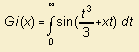# Airy function

## gamma

last updated: 2004-02-24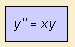is the differential equation which defines the Airy function

The equation has two independent solutions Ai() and Bi(), Ai goes to zero for large x, Bi goes to infinity.
They can be written as integrals (omitting constants):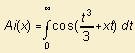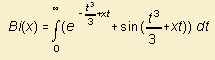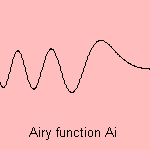The Airy function is found as solution to boundary value problems in quantum mechanics and electromagnetic theory.

Given a potential that increases linearly from left to right. Then the Airy function Ai(x) gives for a particle the quantum-mechanical amplitude. The region at the right side is classically inaccessible, quantum mechanically exponentially damped.

Related Airy functions Gi(x) and Hi(x) have been defined, from the parts of above expression for Bi: